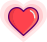### 题目描述

    3   /   9  20    /     15   7

### 不同解法分析

private int max = 1;public int maxDepth(TreeNode root) {    if (root == null) {        return 0;    }    helper(root, 1);    return max;}private void helper(TreeNode root, int currentDepth) {    if (root == null) {        return;    }    max = Math.max(max, currentDepth);    helper(root.left, currentDepth + 1);    helper(root.right, currentDepth + 1);}

public int maxDepth(TreeNode root) {    if (root == null) {        return 0;    }    int left = maxDepth(root.left);    int right = maxDepth(root.right);    return Math.max(left, right) + 1;}

### 思路总结

             老板            /  |             经理 ...  经理         / |     /  |        员工...员工 员工...员工

### 延伸

public int maxDepth(TreeNode root) {    if (root == null) {        return 0;    }    Stack<TreeNode> stackTree = new Stack<>();    Stack<Integer> stackDepth = new Stack<>();    stackTree.push(root);    stackDepth.push(1);    int max = 1;    while (!stackTree.isEmpty()) {        TreeNode curNode = stackTree.pop();        int curDepth = stackDepth.pop();        if (curNode.left != null) {            stackTree.push(curNode.left);            stackDepth.push(curDepth + 1);        }        if (curNode.right != null) {            stackTree.push(curNode.right);            stackDepth.push(curDepth + 1);        }        if (curNode.left == null && curNode.right == null) {            max = Math.max(max, curDepth);        }    }    return max;}

1. 暴力的深度优先搜索

2. 画出/思考出问题和子问题的关系，看有没有重复子问题

3. 如果有重复子问题，考虑增加记忆化的数据结构

4. 据此，思考动态规划的状态和递推方程

5. 实现动态规划

### 总结原 创 热 文 推 荐